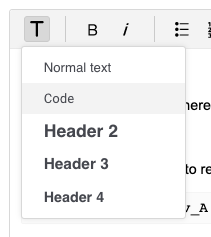•Gurobi Staff

Hi Jose,

The "smatr" dictionary should remain a dictionary since it is data for the problem, not an unknown value that you are solving for.

There are a few syntactical issues with this code. The main one is that you should iterate over the "met" indices within the quicksum function:

model.addConstrs((quicksum(smatr[i,j] * v[j] for i in met) == 0) for j in reactions)

With your data, this produces the following constraints:

\begin{alignat*}2 v_A &= 0 \\4 v_B &= 0 \\2 v_C &= 0 \\v_D &= 0 \\6 v_E &= 0\end{alignat*}

The only solution to this system is the zero vector. Is this what you want?

Eli

•Thank you so much Eli, your answer helps me a lot for the thing I want to maximize is the flux so a zero vector is not much of a help. On a side note, this would be outside of the scope of the original post, I would also ask you how to write chunck of code or Latex in the comments for future posts, could you refer me to some documentation on it.

Jose

•Gurobi Staff

Hi Jose,

To create a block of code, there is a "code" formatting option available when writing a post. Here is a picture:This forum uses MathJax to render math notation. To create the equation system I previously posted (in display mode), I used:

\begin{align*}2 v_A &= 0 \\4 v_B &= 0 \\2 v_C &= 0 \\v_D &= 0 \\6 v_E &= 0\end{align*}

To render math inline, like $$v_A = 0$$, you can use

$$v_A = 0$$

Unfortunately, there is no preview function in this forum, so I often end up editing posts with math in them after submission.

Best,

Eli

•Thanks a lot, Eli, as always very helpful

•Hello Eli,

I tried to write a knapsack constr with the code,

m.addVars(reactions, vtype=GRB.BINARY, name='y')m.addConstr(y.sum(j) <= 1 for j in reactions)

but I get this error

unsupported operand type(s) for -: 'generator' and 'NoneType'

Ultimately I want to write a knpsack constraint like the following?

$\sum (1-y_j)\, \leq \, K$

Cheers

•Gurobi Staff

Hi Jose,

I see two issues with the code:

• You create new model variables using Model.addVars(), but you do not assign the returned tupledict object to a Python variable named y.
• tupledict.sum() is a shortcut method to sum over the values in the tupledict that match the specified pattern. In this case, it is probably more straightforward to write the expression using the quicksum() function.

With a few small changes, we can add the constraint as follows:

# Model.addVars() returns tupledict; assign it to Python variable yy = m.addVars(reactions, vtype=GRB.BINARY, name='y')# Use quicksum() to add constraint# Iterate through set of reactions within quicksum()m.addConstr(quicksum(1 - y[j] for j in reactions) <= K)

Thanks,

Eli

•Hello Eli,

After trying to solve the model I am working on, as you well made it clear the constraint gives me a zero vector, so the solution I get is totally not what I need, I've checked the constraint and I need to have a set of linear equations like the following:

$$(1,A)*v_A + (1,B)*v_B + (1,C)*v_C + (1,D)*v_D + (1,E)*v_E = 0$$

and so on for the rest of the met's, in this case, should I consider

smatr.sum(j)*v[j] == 0 for j in reactionsAnd that would mean to have smatr as a variable?
•Hello Eli,

I've tried this:

for i in met:   m.addConstr((gp.quicksum(smatr[i,j]*v[j] for j in reactions) == 0), name='FBA')

It seems to work as it gives me the equations I want, Now, my question would be, how can I write it in list comprehension?

Thank you so much!

Jose Alexander

•Hello Eli,

I am sorry to bother again, but I am trying to solve an FBA, and after I get the solution is still different from the example I am basing on, so I tried to add as a constraint the solution to check where my program falls short, let's say I am interested in a solution where the flux of $$v_D$$ is equal to a number, I added the constraint as follows, but I get an error, is there a way to add said constraint?

m.addConstr(v == 10)
•Gurobi Staff

Hi Jose,

You can add your constraints in a single statement using the Model.addConstrs() method. Instead of passing a single constraint to the method, you pass a generator expression for an entire set of constraints. In this case, the syntax is:

m.addConstrs((gp.quicksum(smatr[i,j]*v[j] for j in reactions) == 0 for i in met), name='FBA')

Do you receive a KeyError when you try to add the constraint v == 10? In your original post, you create a $$v$$ variable for every reaction:

v = m.addVars(reactions, obj=biomass)

The Model.addVars() method returns a tupledict object with keys indexed by the elements of the reactions set. Thus, you can access the $$v_D$$ variable by using the corresponding reaction as the key:

m.addConstr(v['D'] == 10)

If you haven't done so already, I recommend writing the model to an LP file when debugging. You can do this with the Model.write() method. E.g.:

m.write('fba.lp')

The LP file format is human-readable, which makes it easy to visually inspecting a model. You may also want to add names to your variables if you do this.

Thanks,

Eli